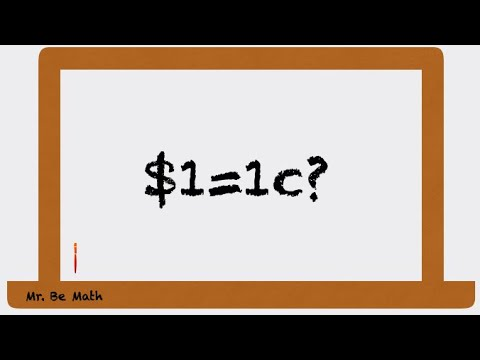Anonymous
Anonymous asked in Science & MathematicsMathematics · 7 days ago

# Is -5 squared 25 or -25?

The problem only asks to find -5 squared, so I think it would be 25 because -5 times -5 is 25. However, my friend thinks -5 squared is -25. She thinks this because since it doesn't have parenthesis it would be mean the opposite of 5 squared. Therefore 5 times 5 is 25 and the opposite of that is -25. Who is right?

Relevance
• Anonymous
3 days ago

A negative times a negative number would always equal to a positive one but the answer could vary given the parenthesis. If somehow the value is (-5)² then the answer would still be 25, but if given, -(5)², one's task is to firstly solve and square it first before combining it with a negative value, which, in this case, the answer should be -25.

•Login to reply the answers
• It's not telling you to take -5 and square it.

It's telling you to take 5 and square it, then take the negative.

-5² = -5·5 = -25

(-5)² = (-5)(-5) = 25

•Login to reply the answers
• It depends, you should state whether a parenthesis is present or not. For example, -5^2 would be -25, while (-5)^2 would be 25. So, what the problem asks is not really specific, so I would have to say both you and your friend are correct

•Login to reply the answers
• 25.  5i squared would be -25.

•Login to reply the answers
• I can see why your friend is confused. parenthesis make the operation clear. When you ask me what -5 squared is, you can write it as (-5)^2, which, as you said, is 25.

If you were to write it as -(5)^2, then yeah it is -25.

•Login to reply the answers
• Given i^2 = -1 by definition, we have -5 = -1*5 = i^2 * 5; so that (i^2 * 5)^2 = i^4 * 5^2 = (-1)*(-1) * 5^2 = +1* 25 = 25  QED.

•Login to reply the answers
• Its better think about the concept of squaring: (-5)² = (-5). (- 5). But if you have any algebraic expression written -5 ^ 2 then the result will be: -5² = (-1) (5) (5) = - 25. Some small things can lead to confusion in mathematics.•Login to reply the answers
• as others have said, english is ambiguous.

(–5)² = 25

–(5)² = –25

•Login to reply the answers
• The question is ambiguous.  Obviously parentheses would clear up the ambiguity.

If you see the expression -x^2, most people agree that equals -1 times (x^2).  For example y = -x^2 is the upside down parabola y = -(x^2).

Therefore, I am going with -25.

•Login to reply the answers
• (-5)(-5) = 25  << this is the assumed meaning

- (5)(5) = -25  << If you wanted this, say the negative "of" 5 squared.

Using English for math problems can be problematic unless specific.

Even WA says it's 25Source(s): But you knew this already
•Login to reply the answers